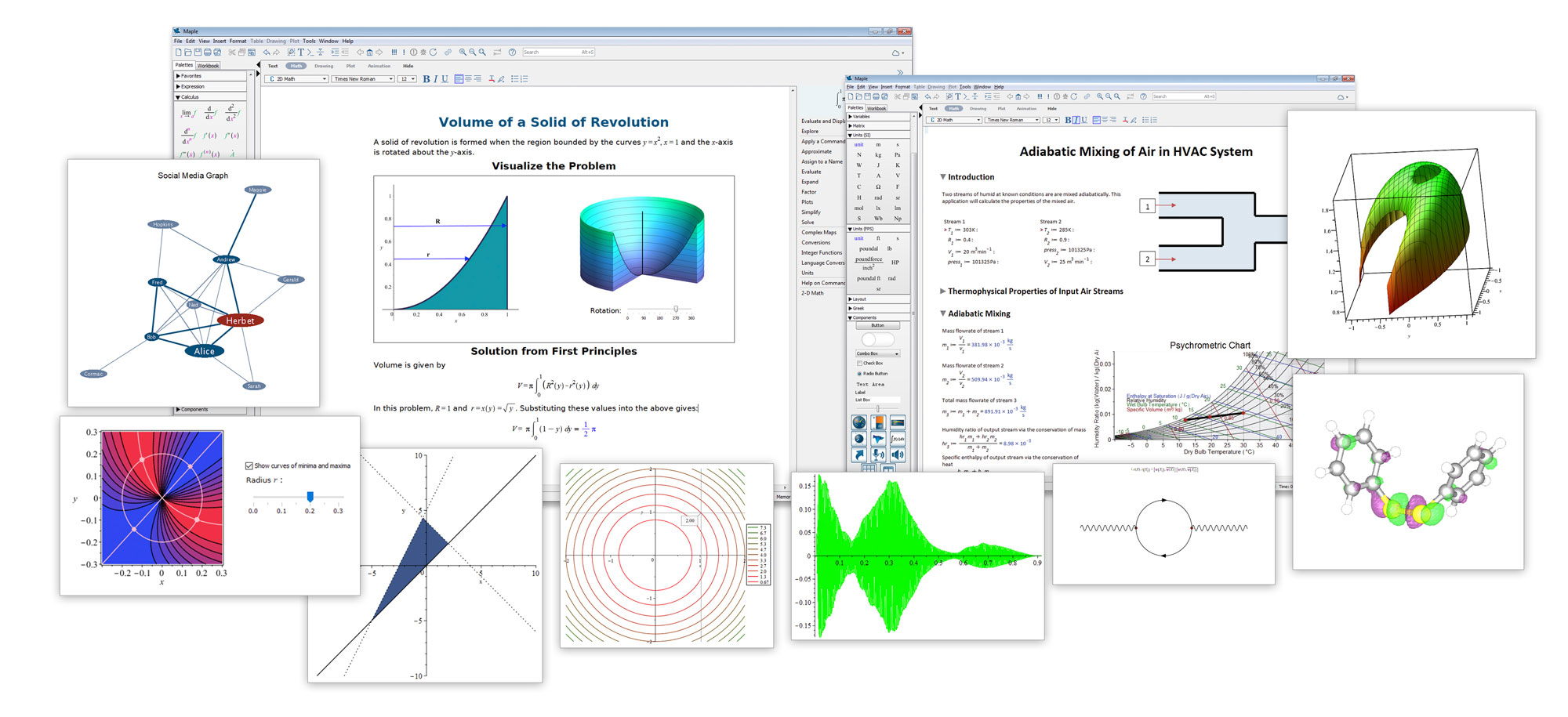# Day 1: The Basics

The first day delivers the fundamental skills you need to be a successful Maple user. A series of exercises ensure attendees acquire and retain the skills demonstrated throughout the day.

### Navigating the help menu and accessing support resources

#### Entering and editing math expressions

• Using the palettes and the keyboard
• Simple calculations and numerical formatting
• Equation labels to reference and manipulate results

### Working with variables and functions

• Creating variables and functions
• Scope and evaluation precedence
• Piecewise functions
• Suppressing/displaying intermediate results
• Clearing memory space

### Entering and editing text

• Customizing text formats and styles
• Switching between math and text

### Formatting a document

• Inserting whitespace and sections/subsections
• Importing/inserting pictures, sketching canvas

• Point-and-click differentiation, integration, matrix manipulation, plotting

### Basic Maple commands

• Symbolic and numeric solution of equations
• Differentiation, integration
• Command completion
• Introduction to Maple packages

### Basic plotting

• Plotting expressions and functions
• Formatting and overlaying plots

### Basic data structures

• Expression sequences, lists, sets, arrays
• Manipulating and referencing data structures

### Introductory Maple programming

• Choosing between document or worksheet mode
• Creating simple procedures using looping and conditional statements

# Day 2: Intermediate Techniques

The second day builds on the earlier topics, and helps you build compelling Maple applications. Given the interests of the attendees, certain subjects may be emphasized or not covered. Please discuss your needs with your trainer before the session.

### Solving Differential Equations

• Entering odes in different notations
• Symbolic and numeric solutions
• Ode assistant and command-based solutions
• Plotting and visualizing solutions

### Matrix and Vector Computations

• Data structures: Matrix, Vector
• Creating, manipulating and referencing matrices and vectors
• Matrix math and linear algebra (for example, solving linear systems)
Data Analysis
• Importing and plotting data (with context-sensitive menus and commands)
• Curve fitting, single-variable regression
• Data statistics (standard deviation, variation, R-squared, etc.)

### Dynamic Systems

• Creating dynamic system objects (transfer functions, differential equations, state space)
• Converting between different representations (context-sensitive menus and commands)
• Bode/Phase/Nyquist/Root-locus plots
• Discretizing transfer functions

### Optimization

• Finding minima/maxima
• Least-squares and multivariable regression techniques for model fitting
• Linear programming
• Global optimization (requires the Maple Global Optimization Toolbox)

### Creating GUIs and interactive documents

• Sliders, buttons, etc.
• Fine-tuning the look of an interactive document
Scripting behavior
• Deploying to the web (requires MapleNet™)